# Semi-modular lattice

(diff) ← Older revision | Latest revision (diff) | Newer revision → (diff)
A lattice in which the modularity relation is symmetric, i.e.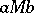implies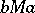for any lattice elements. The modularity relation here is defined as follows: Two elementsandare said to constitute a modular pair, in symbols, iffor any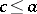. A lattice in which every pair of elements is modular is called a modular lattice or a Dedekind lattice.
A lattice of finite length is a semi-modular lattice if and only if it satisfies the covering condition: Ifandcover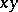, then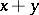coversand(see Covering element). In any semi-modular lattice of finite length one has the Jordan–Dedekind chain condition (all maximal chains between two fixed elements are of the same length; this makes it possible to develop a theory of dimension in such lattices. A semi-modular lattice of finite length is a relatively complemented lattice if and only if each of its elements is a union of atoms. Such lattices are known as geometric lattices. An important class of semi-modular lattices is that of the "nearly geometric" matroid lattices (see ). Every finite lattice is isomorphic to a sublattice of a finite semi-modular lattice. The class of semi-modular lattices is not closed under taking homomorphic images.